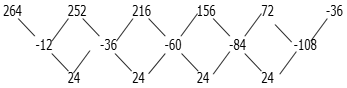# Important Number Series Questions For Quantitative Aptitude Section For IBPS Clerk Prelims 2017

Dear Bankersdaily Aspirant,

The IBPS CLERK PRELIMS EXAM 2017 is over partially and we still have two days of exam remaining. SO if you check the review and analysis of IBPS CLERK prelims exam 2017 and the various slots of the exam, you can see that 5 questions are asked from the Missing Number series in the Quantitative Aptitude section.

So we can expect the same in the forthcoming batches too, here were are providing some questions to solve to entice your preparations for the IBPS CLERK PRELIMS EXAM 2017. You can get surely 5 marks which could boost your score to a different level.

Candidates can check the review of the IBPS CLERK PRELIMS EXAM 2017 from the given links which consists of all the reviews of all the slots. So this will be a great source of info and for your preparations, if you have the IBPS CLERK PRELIMS EXAM 2017 in the forthcoming days.

You can solve the questions given below to gain some practice for the IBPS CLERK PRELIMS EXAM 2017.

Q (1 – 5 ) Find the missing number in the following number series

1)  3      9    23    97    ___     2881

a) 438

b) 479

c) 379

d) 437

e) None of these

2) 24    12   __   18     36    90   270

a) 12

b) 13

c) 16

d) 18

e) 15

3) 264   252   216   156   72   __

a) 36

b) 24

c) -36

d) -24

d) 10

4) 15   31   66   141   __   621

a) 298

b) 278

c) 398

d) 128

e) None of these

5) 1   1.5   3.5   __   44   357

a) 9.5

b) 10.5

c) 11

d) 10

e) None of these

Q 6-10) What will come in place of blank space in the given number series?

6) __, 174, 116, 58, 23.2

a) 185

b) 188

c) 183

d) 182

e) None of these

7) 48,  72,  90,  __,  118.125

a) 106

b) 105

c) 104

d) 103

e) None of these

8) 529,  841,  961,  1369,  __

a) 1525

b) 1681

c) 1623

d) 1728

e) None of these

9) 375,  __,  407,  437,  479

a) 387

b) 388

c) 389

d) 390

e) None of these

10) 3375,  4096,  4913,  5832,  __

a) 6889

b) 6879

c) 6858

d) 6859

e) None of these

You can check the answers of the previous questions from the one below. So if you got all the questions correct, you are in great form and keep going with the preparations for the IBPS CLERK PRELIMINARY EXAMINATION 2017 and also for the RBI ASSISTANT MAINS EXAMINATION 2017.

Q.1) b

3×2+3=9

9×3-4=23

23×4+5=97

97×5-6=479

479×6+7=2881

Q.2) a

24×0.5=12

12×1=12

12×1.5=18

18×2=36

36×2.5=90

90×3=270

Q.3) cQ.4) a

15×2+12=31

31×2+22=66

66×2+32=141

141×2+42=298

298×2+52=621

Q.5) d

1×0.5+1=1.5

1.5×1+2=3.5

3.5×2+3=10

10×4+4=44

44×8+5=357

Q.6) e

÷1,    ÷1.5, ÷2,    ÷2.5

Q.7) b

×3÷2,                   ×5÷4,                   ×7÷6,                   ×9÷8

Q.8) b

232, 292, 312, 372, 412

Q.9) a

+3×4,                   +4×5,                   +5×6,                   +6×7

Q.10) d

153, 163, 173, 183, 193